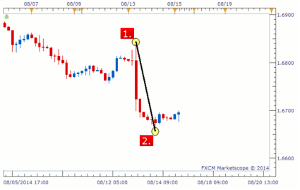# The &lsquo;Magic&rsquo; Behind Fibonacci

08/26/2014 6:00 am EST

Focus: STRATEGIES

Rob Pasche of DailyFX.com discusses the magic behind the Fibonacci retracement tool, including where and how to place it on the charts and how it can, at times, create successful trades.

Today, we take a look at how to use the Fibonacci retracement tool on our charts and uncover the magic that can make it work.

Where to Draw the Fibonacci Retracement?

Unfortunately, Fibonacci will not automatically appear on your chart like a moving average or RSI will. We must draw on the chart ourselves where we would like the Fibonacci to go. But where exactly is that? For starters, we need to identify an extended move up or down on our chart. In other words, a swing low followed by a defined high or a swing high followed by a defined low. Below, we have an example of a swing high (labeled 1) followed by a swing low (labeled 2).

Learn Forex: Drawing Fibonacci Retracement on a ChartCreated using Marketscope 2.0 charting package
Click to Enlarge

The Fibonacci Retracement requires us to mark these two points, left-to-right.

What Do the Fibonacci Lines Mean?

After drawing the two data points, we see three blue lines appear between the high and the low: 0.382, 0.500, and 0.618. These numbers represent how far price could retrace in percentage terms. So if price rose back to the 0.382 level that would be a retracement of 38.2% of the original move. If price rose to the 0.500 level, that would be a retracement of 50% of the original move. If price rose to the 0.618 level, that would be a retracement of 61.8% of the original move.

We can see these levels labeled in the chart below as potential resistance levels. These are price levels that price could bounce off of.

Learn Forex: Fibonacci Retracement Projecting Potential Resistance Levels

There is no exact science to determine which level price will bounce or if price will bounce off any of the levels at all. But time and time again, we will see price bounce off of one of these three retracement levels created by using Fibonacci.

Why Does Fibonacci Seem to Work?

This is probably the most popular question I get regarding Fibonacci. Why does price bounce off these levels? What is the magic that causes this? In my opinion, there is no magic involved in it at all. What causes price to sometimes react around these levels is due to the fact that many people are watching these exact same levels.

If we have a large number of traders all looking at the same levels for a turnaround in price, they could create a cluster of orders that causes price to actually turn around at that level. It won’t always be that cut and dry, but that is the gist of using this type of tool. We are expecting other traders to be looking at these levels and cause price to react at these levels. It is self-fulfilling.

Learn Forex: Price Bouncing Off Fibonacci’s 38.2% Retracement Level

The chart above shows the outcome of our example. Price retraced up to the 38.2% retracement level and stalled for a while before moving back in the direction of the original move. Traders that traded the potential bounce off of this level had a very successful trade without having to take on a lot of risk if they used a tight stop.

Fibonacci Fraternity

So hopefully this article has unlocked the mystery behind Fibonacci by showing how to draw this tool on a chart and by teaching why the Fibonacci can, at times, create successful trades. You are now on your way to joining the Fibonacci club.

By Rob Pasche, Trading Instructor, DailyFX.com